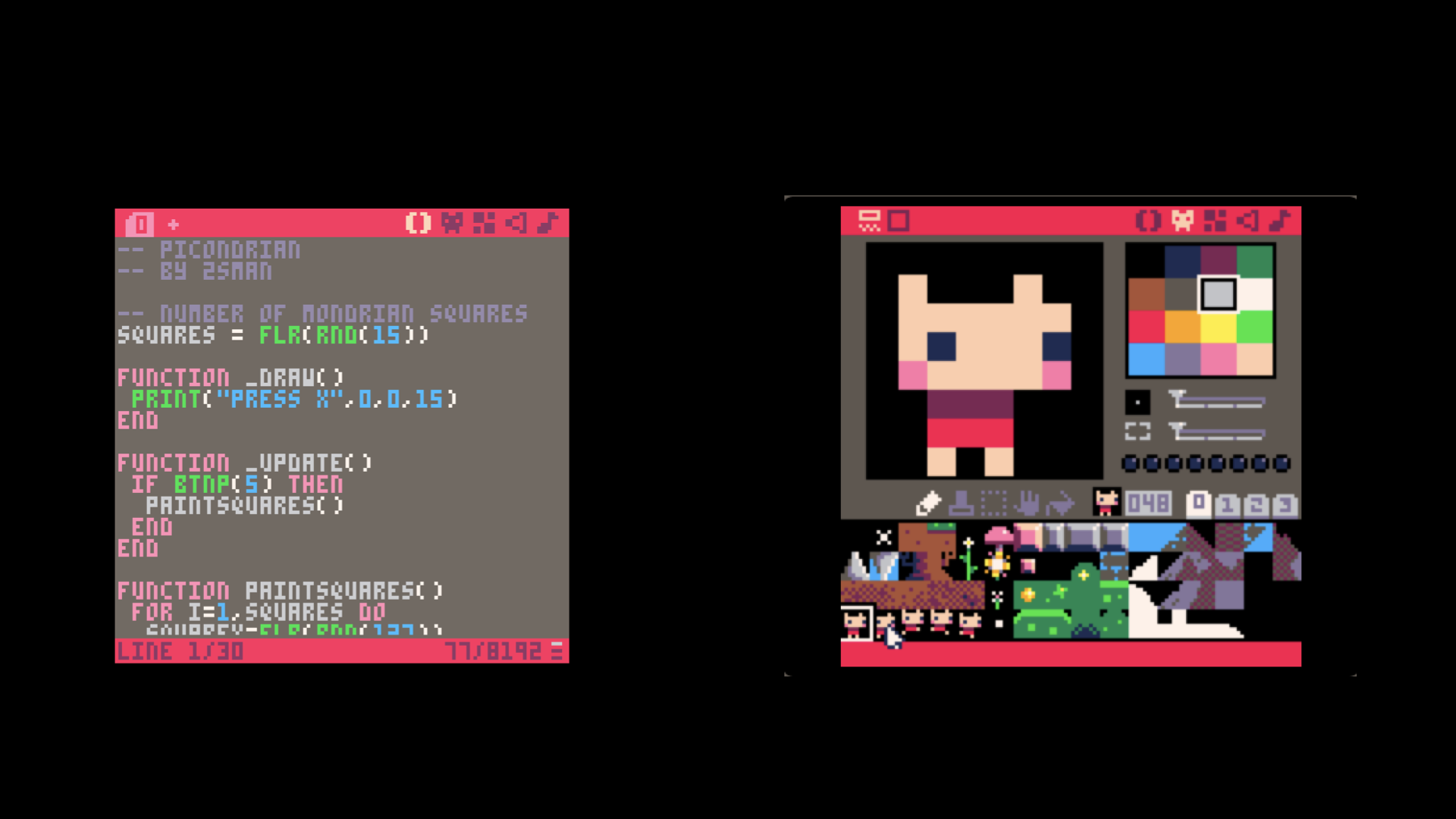# Pico8 Lua cheatsheet

22 Oct 2017``````-- use two hyphens like this to ignore everything until the end of the line
--[[ multi-line
``````

# Types and assignment

Types in Lua are numbers, strings, booleans and tables;

``````NUM = 12/100
S = "THIS IS A STRING"
B = FALSE
T = {1,2,3}
``````

# Conditionals

``````IF NOT B THEN
PRINT("B IS FALSE")
ELSE
PRINT("B IS NOT FALSE")
END

-- with ELSE-IF

IF X==0 THEN
PRINT("X IS 0")
ELSEIF X < 0 THEN
PRINT("X IS NEGATIVE")
ELSE
PRINT("IF NONE OF THE ABOVE")
END
``````

`IF (4 == 4) THEN PRINT("EQUAL") END` `IF (4 != 3) THEN PRINT("NOT EQUAL") END -- note that != can also can be written as ~=`

# LOOPS

• starts with 1, NOT 0
``````FOR X=1,5 DO
PRINT(X)
END

FOR X=1,10,3 DO PRINT(X) END   -- 1,4,7,10
``````

# FUNCTIONS

``````Y=0
FUNCTION PLUSONE(X)
LOCAL Y = X+1
RETURN Y
END
PRINT(PLUSONE(2)) -- 3
PRINT(Y) 	  -- 0
``````

# TABLES

• In Lua, tables are a collection of key-value pairs where the key and value types can both be mixed. They can be used as arrays by indexing them with integers.
``````A={} -- create an empty table
A = "BLAH"
A = 42
A["FOO"] = {1,2,3}

-- Arrays use 1-based indexing by default

A = {11,12,13,14}
PRINT(A) -- 12

-- size of a table indexed with sequential 1-based integers

PRINT(#A) -- 4

-- Indexes that are strings can be written using dot notation

PLAYER = {}
PLAYER.X = 2 -- equivalent to PLAYER["X"]
PLAYER.Y = 3
``````

# GENERAL PICO8 CARTRIDGE NOTES

• To generate a preview image saved with the cart, run the program first and press F7 to grab whatever is on the screen.
• The first two lines of the program starting with ‘–’ are also drawn to the cart’s label. e.g.
``````-- OCEAN DIVER LEGENDS
-- BY LOOPY
``````

``````folder - open the carts folder in host operating system